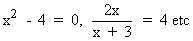Home MonkeyNotes Printable Notes Digital Library Study Guides Study Smart Parents Tips College Planning Test Prep Fun Zone Help / FAQ How to Cite New Title Request

 CHAPTER 7 : EQUATIONS, INEQUATIONS, GRAPHING AND ABSOLUTE VALUE 7.1 Definition An algebraic equation is a statement that two algebraic expression are equal. Letters involved in these equations are variable or unknowns. If the equality is true for certain values of unknowns involved in equations, then these equations are called conditional equations. For examples,The values of unknowns of an equation, satisfying that equation, are known as solutions of that equation. While solving a problem, we first reduce it to an equation then try to find the value of the unknown in the equation; this is known as "solving the equation" An equation is not altered if we (1) add equal numbers to each side; (2) subtract equal numbers from each side; (3) multiply each side by equal numbers; (4) divide each side by equal numbers. Solving Simple Equations : Example Solve x - 2 = 6 Solution : Adding 2 to each side, we have x = 8 Example Solve x + 3 = 5 Solution : Subtracting 3 from each side, we have x = 2 Example SolveSolution : Multiplying each side by 3, we have x = 15 Example Solve 3x = 6 Solution : Dividing each side by 3, we have x = 2 Example Solve 7x - 2 = 3x + 6 Solution : Adding 2 to each side; 7x = 3x + 8       Subtracting 3x from each side; 4x = 8       Dividing by 4 to each side; x = 2 Index 7.1 Definition7.2 Simultaneous Equations7.3 Inequations (Inequalities)7.4 Absolute Values Chapter 8
 Search: All Products Books Popular Music Classical Music Video DVD Toys & Games Electronics Software Tools & Hardware Outdoor Living Kitchen & Housewares Camera & Photo Cell Phones Keywords: